# The Development of Mathematical Reasoning, part 2

Uncategorized Jun 04, 2020

In my last blog, I wrote about the Development of Mathematical Reasoning.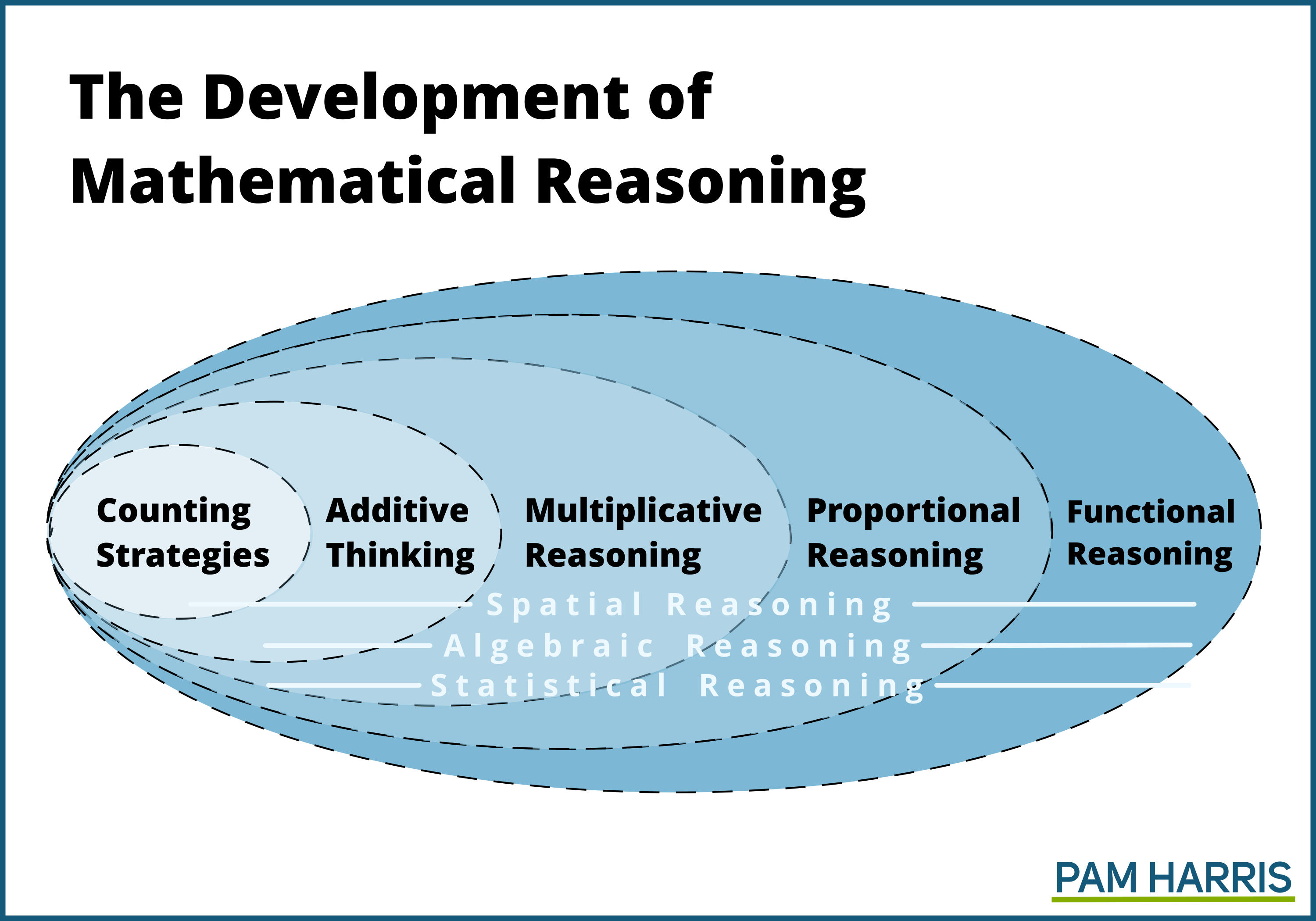The Development of Mathematical Reasoning graphic displays an important mathematical hierarchy of progressive relationships, ways of thinking and reasoning that build on each other. Each of these ways of thinking and reasoning is more sophisticated than the prior. Each deals with more simultaneity – more things to deal with at the same time. Each of these ways of thinking and reasoning can be thought of as structuring and schematizing — ways of viewing and dealing with mathematics. For lack of better terms, I’ll call them levels or domains of reasoning.

### Students need to develop each level of reasoning so that they can build on it for the next level.

It is important for students to develop counting strategies because counting is essential in the development of additive thinking.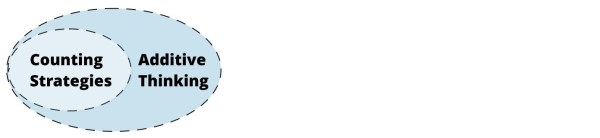And additive thinking is essential for students to develop multiplicative reasoning so that they can use multiplicative strategies.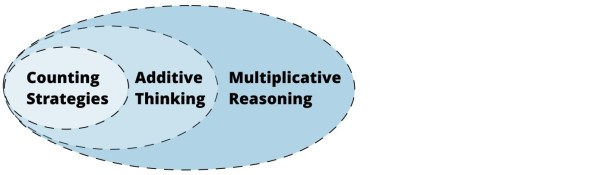Without multiplicative reasoning, it is impossible to develop proportional reasoning, which is the land of fractions, ratios, proportions, and percents.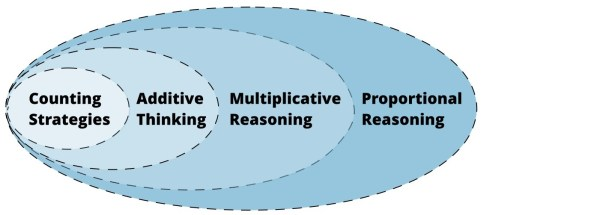Proportional reasoning then allows students to reason about complex function relationships as covariation.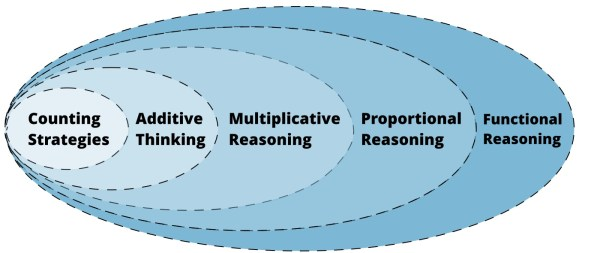It is not about getting answers to types of problems, but it is about the development of ways of thinking and reasoning.

When we, as mathematics teachers, understand this hierarchy then we treat teaching mathematics as more than helping students get answers. We treat teaching mathematics as helping students develop mental relationships and connections, literally changing the way students think. It’s about mentoring students in the act of mathematizing in increasingly sophisticated ways.

It’s about mentoring students in the act of mathematizing in increasingly sophisticated ways.

As a culture, we have misunderstood this progression. We have seen textbooks and standards that call for students to add and subtract, then to multiply and divide, followed by operating with fractions, decimals, percents and proportions, and finally algebra and functions. But rather than develop students who think in those ways, we have allowed mere answer getting. We have allowed students to rote memorize facts and mimic procedures to find answers to problems in each of the levels. This often looks like students getting answers to problems that live in each level by using reasoning from less sophisticated levels. If the goal is to help students develop as multiplicative thinkers, then accepting answers found using additive thinking is counter-productive. The goal is not answers. The goal is development. We don’t need students who can just answer a multiplication question, we need students who can reason multiplicatively.

We don’t need students who can just answer a multiplication question, we need students who can reason multiplicatively.

I submit that is in large part because we have not understood this development progression and thus we have allowed students to get answers to problems using reasoning from previous levels (less sophisticated) and therefore those students cannot move on to develop the reasoning in the next level in the progression. Students "look" successful at a level, but do not have the mental schema to understand the next level.

At each stage, we cannot be complacent with students solving problems, getting answers, using the reasoning of the level before. Instead, we must help students develop more sophisticated thought so that they can continue to develop more sophisticated notions of mathematics. Success in each level is dependent on the previous and is built using the previous. But success is defined as developing (fill in the blank) reasoning, not as simply getting answers to (fill in the blank) problems.

But success is defined as developing (fill in the blank) reasoning, not as simply getting answers to (fill in the blank) problems.

It is important that teachers of mathematics develop their own schema for each domain, understand deeply each domain, how each mathematization leads to the next level of sophisticated thought and how we as teachers can hinder or support students’ development.

What are some of the ramifications? Here are a few examples.

##### Students should be learning additive reasoning, but:

Students are taught that addition is synonymous with the traditional algorithm: line numbers up, add each column from right to left, small to big. If students use the traditional addition algorithm, they can, at worst, be summing each of the columns by counting by 1s, thus getting addition answers using a counting strategy. With the algorithm, they can, at best, be using additive thinking but only for single digits. They never get a chance to think about the numbers 38 and 49, because the algorithm has them thinking about 8 + 9 and 3 + 4 + 1.

We need to do work with students besides using the traditional algorithm. Students should be able to reason in bigger jumps of numbers, that 38 + 49 = 40 + 47 or 38 + 49 = 37 + 50. That 347 + 299 = 347 + 300 – 1 = 647 – 1 = 646. That 4.95 + 1.47 = 5 + 1.42 = 6.42.  These are example of the kinds of strategies students can develop in 2nd-5th grade—this is developing additive thinking.

One way to view the differences of solving addition problems between using counting strategies and additive thinking is the following graphic. Counting strategies means that students are counting one by one. Additive thinking means that students are using fewer, bigger jumps of numbers.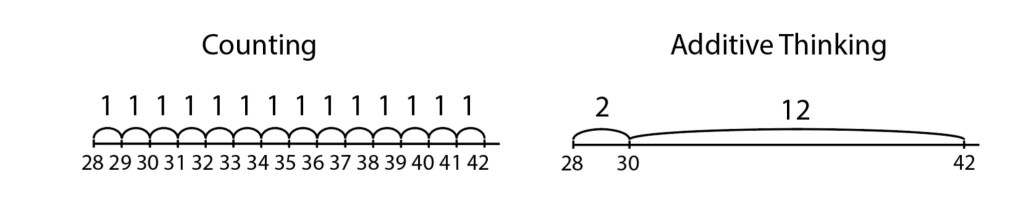##### Students should be learning multiplicative reasoning, but:

Students are taught that multiplication is synonymous with memorizing single-digit facts and with the traditional algorithm: line numbers up, multiply each column from right to left, small to big. If students know only the traditional multiplication algorithm, we see often that students are skip counting to find the individual steps. Skip counting is additive thinking. Thus students are getting answers to multiplication questions but only thinking additively. Or, slightly better are students who are reasoning multiplicatively about the single digit facts. But using the algorithm, students will not reason about more than single digit facts. Students need to be able to reason in bigger chunks of numbers. For example, students using the algorithm think about 99 × 47 as 7 × 9, write down the 3, carry the 6, 7 × 9 and add 6, then drop the magic 0 and do the second row.

But students could be thinking about 99 × 47 as one hundred 47s subtract 47. Or they could be reasoning about 25 × 36 as 25 × 36 = 50 × 18 = 100 × 9. Or students could use factoring flexibly to find 45 × 16 as (9 × 5) × (2 × 8) = 9 × (10) × 8 = 720. These are examples of the kind of strategies students should learn in 3rd, 4th, and 5th grade—this is developing multiplicative thinking.

One way to view the differences of solving multiplication problems between using counting strategies, additive thinking, and multiplicative thinking is the following graphic. Counting strategies mean that students are counting all of the things in each set. Additive thinking means that students are skip counting, one group at a time. Multiplicative thinking means that students are choosing fewer, larger chunks.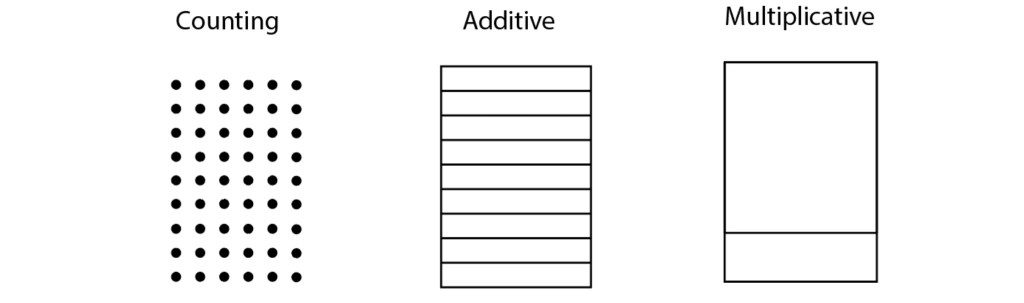##### Students should be learning proportional reasoning, but:

Students are taught to cross multiply and divide to solve proportions. Many middle school teachers ask me why we shouldn’t teach students to cross multiply and divide to solve proportions. Consider the operations involved in cross multiply and divide — they are multiplicative operations. So, instead of students learning to reason proportionally, they are using the reasoning in the level before, a less sophisticated level. And if they are using algorithms to do the cross multiplying and dividing, they are at best thinking in terms of single digits. And on top of that, then students mix the cross multiply and divide rule up with every other fraction rule (Is this where we invert and multiply, or find common denominators, or multiply straight across or cross cancel — which isn’t even a thing!). So we end up with students who get answers to proportions if they remember which rule to apply, but the rule is based on a less sophisticated set of reasoning.

Instead we need students who can look at a proportion like 8:3 = 42.4:𝒙 and think, what does it take for me to get from 8 to 42.4? Then scale in tandem to find what 3 scales to, as in this graphic.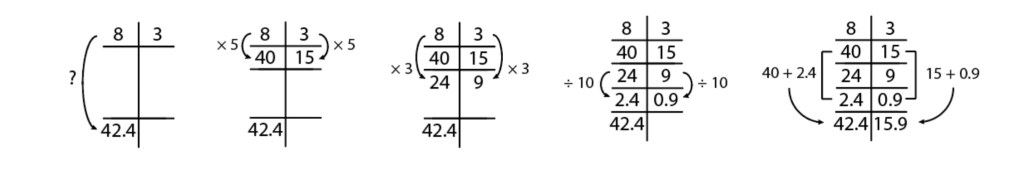This scaling in tandem uses multiplicative thinking inside of proportional reasoning, because qualities are scaling in tandem, according to the given ratio.

##### Students should be learning functional, covariation reasoning, but:

Students are taught to subtract, subtract, divide to find slope and then plug values into formulas to find the equations of lines. And sometimes the subtract, subtract happens by counting tick marks on graphs. All the way back to counting! Students aren’t reasoning about linearity, they are minus-ing and plugging.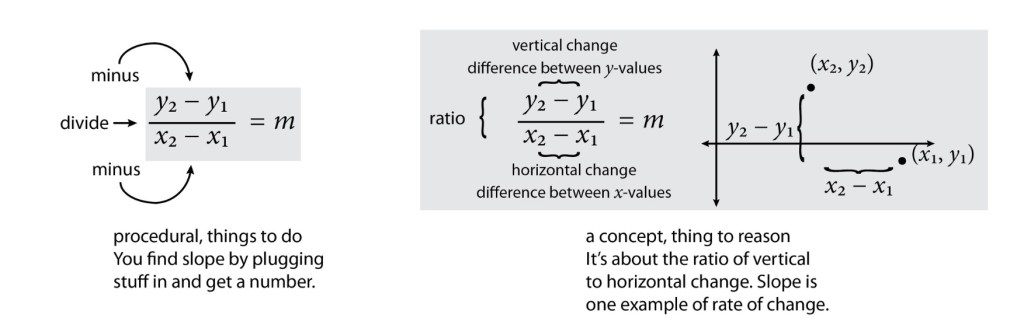In function reasoning, we need students who can reason about ratios as the relationship between quantities that vary and are linked, and apply those understandings as dilations to infinite sets of points that follow a functional rule. Students can use motion detectors to develop notions about how motion affects graphs of (elapsed time, distance). They can reason about direction, rate, and starting point to understand that “where you end up = starting point + rate (time)” or y = begin + move(x) and transition to y = b + mx. Students can then use reasoning to find the equation of a line where they know that the person is walking at a rate of 2 meters per second and at three seconds were 9 feet away.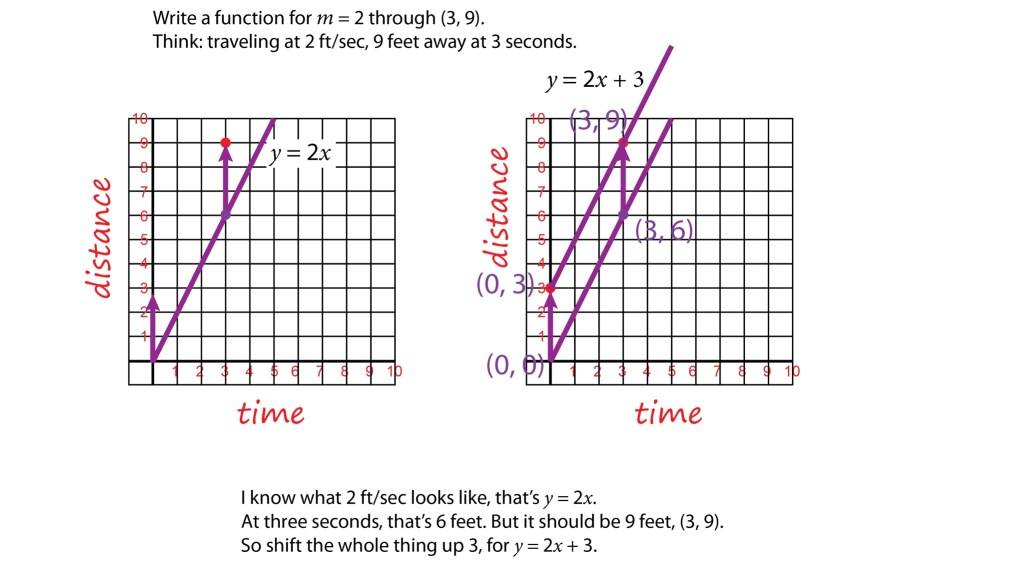##### Using the Development to Influence Teaching Decisions

If teachers understand this development, then they will do the work to engage students’ brains in developing increasingly sophisticated ways of figuring. Teachers and students will not be satisfied with answers, but focus on the reasoning involved, looking for clever, efficient strategies, and celebrating clever thinking — not mindless application of the same tedious general solution every time. Teachers will not focus on rote mathematics, but on mentoring mathematicians.

If traditional arithmetic algorithms come too soon, are emphasized too much, or are taught as "the thing to do", students will complacently repeat steps and get answers, but will be either stuck using reasoning from the level(s) before or, at best, using reasoning about mere single digits. This kind of reasoning is not sufficient to own that level, therefore this kind of reasoning is not helpful to build the next level.

So, where do you fit in the development? How can you work to help your students development the thinking and reasoning, the schema and structuring for that kind of thinking? How can you delay traditional algorithms? How can you emphasize reasoning over rote mimicry? How can you celebrate students wherever they are while always working to help them develop further?

How can you celebrate students wherever they are while always working to help them develop further?

If you haven't already, check out this out! How are your students reasoning? Are you curious? To help you, I’ve written a free, one page questionnaire to help you find out.Close